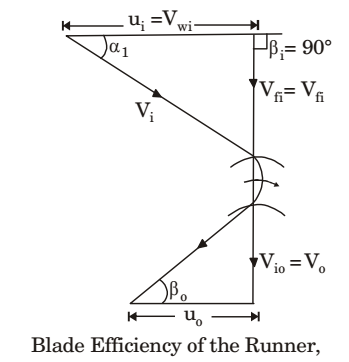## Fluid Mechanics and Hydraulic Machinery Miscellaneous

#### Fluid Mechanics and Hydraulic Machinery

1. A model of a hydraulic turbine is tested at a head of 1/4th of that under which the full scale turbine works. The diameter of the model is half of that of the full scale turbine. If N is the RPM of the full scale turbine, then the RPM of the model will be

1. Given data:

 Model: Hm = Hp ;Dm = Dp 4 9

Nm = ?
Prototype (Full scale turbine)
Np = N
 CH = H (ND)2

(CH)model = (CH)prototype
 Hm = Hp Nm²Dm² Np²Dp²

 Hp/4 = Hp Nm² ×(Dp²/4) Np²Dp²

Nm = N

##### Correct Option: C

Given data:

 Model: Hm = Hp ;Dm = Dp 4 9

Nm = ?
Prototype (Full scale turbine)
Np = N
 CH = H (ND)2

(CH)model = (CH)prototype
 Hm = Hp Nm²Dm² Np²Dp²

 Hp/4 = Hp Nm² ×(Dp²/4) Np²Dp²

Nm = N

1. The inlet angle of runner blades of a Francis turbine is 90°. The blades are so shaped that the tangential component of velocity at blade outlet is zero. The flow velocity remains constant through out the blade passage and is equal to half of the blade velocity at runner inlet. The blade efficiency of the runner is

1. Given data:
Bi = 90°
Vwo = 0

 Vfi = Vfo = Ui 2ηH = Vwiui = U1² ∴  Vwi = ui gH gH

By energy balance equation,
 ρQgH = ρQVwiUi + ρQV0² 2

 gH = Ui² + Vfo² Ui² + Ui² 2 8

 gH = 9 Ui² 8

 ∴  ηH = Ui² = 8 = 0.8888 (9/8)Ui² 9

= 88.88% ≈ 98%

##### Correct Option: D

Given data:
Bi = 90°
Vwo = 0

 Vfi = Vfo = Ui 2ηH = Vwiui = U1² ∴  Vwi = ui gH gH

By energy balance equation,
 ρQgH = ρQVwiUi + ρQV0² 2

 gH = Ui² + Vfo² Ui² + Ui² 2 8

 gH = 9 Ui² 8

 ∴  ηH = Ui² = 8 = 0.8888 (9/8)Ui² 9

= 88.88% ≈ 98%

1. A large hydraulic turbine is to generate 300 kW at 1000 rpm under a head of 40 m. For initial testing, a 1: 4 scale model of the turbine operates under a head of 10 m. The power generated by the model (in kW)will be

1.  PM = Pp =Nm3Dm5 Np Dp

 = 3002000315 = 2.34 kW 1000 5

##### Correct Option: A

 PM = Pp =Nm3Dm5 Np Dp

 = 3002000315 = 2.34 kW 1000 5

1. The head loss for a laminar incompressible flow through a horizontal circular pipe is h1. Pipe length and fluid remaining the same, if the average flow velocity doubles and the pipe diameter reduces to half its previous value, the head loss is h2. The ratio h2 /h1. is

1.  Head loss, h ∝ uavg D2

 ∴ h2 =D12 uavg,2 = 22 = 2 h1 D2 uavg,1

 h2 = 8 h1

##### Correct Option: C

 Head loss, h ∝ uavg D2

 ∴ h2 =D12 uavg,2 = 22 = 2 h1 D2 uavg,1

 h2 = 8 h1

1. Three parallel pipes connected at the two ends have flow-rates Q1, Q2 and Q3 respectively, and the corresponding frictional head losses are hL1, hL2 and hL3 respectively. The correct expressions for total flow rate (Q) and frictional head loss across the two ends (hL) are

1. Total flow rate Q = Q1 + Q2 + Q3
Head loss h = hL1 = hL2 = hL3

##### Correct Option: B

Total flow rate Q = Q1 + Q2 + Q3
Head loss h = hL1 = hL2 = hL3